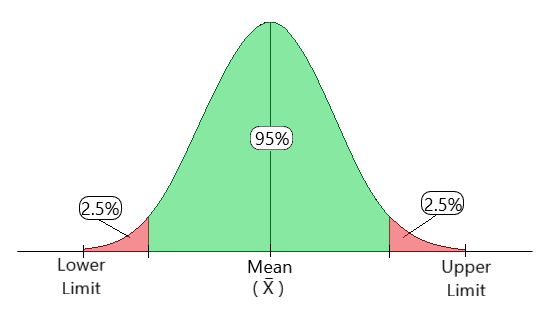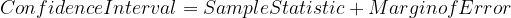# Confidence Interval

• Last Updated : 26 Nov, 2020

Prerequisites: t-test , z-test

In simple terms, Confidence Interval is a range where we are certain that true value exists. The selection of a confidence level for an interval determines the probability that the confidence interval will contain the true parameter value. This range of values is generally used to deal with population-based data, extracting specific, valuable information with a certain amount of confidence, hence the term ‘Confidence Interval’.

Attention reader! Don’t stop learning now. Get hold of all the important Machine Learning Concepts with the Machine Learning Foundation Course at a student-friendly price and become industry ready.

Fig 1. Shows how a confidence interval generally looks like.Fig 1: Confidence Interval Illustration

Confidence Level:

The confidence level describes the uncertainty associated with a sampling method.

Suppose we used the same sampling method (say sample mean) to compute a different interval estimate for each sample. Some interval estimates would include the true population parameter and some would not.

A 90% confidence level means that we would expect 90% of the interval estimates to include the population parameter. A 95% confidence level means that 95% of the intervals would include the population parameter.

For example, let’s suppose you were surveying an average height of men in a particular city. To find that, you set a 95% confidence level and find that the 95% confidence interval is (168,182). That means if you repeated this over and over, 95 percent of the time the height of a man would fall somewhere between 168 cm and 182 cm.

Constructing a Confidence Interval:

Constructing a confidence interval involves 4 steps.

Step 1: Identify the sample problem. Choose the statistic (like sample mean, etc) that
you will use to estimate population parameter.

Step 2: Select a confidence level. (Usually, it is 90%, 95% or 99%)

Step 3: Find the margin of error. (Usually given) If not given, use the following formula:-
Margin of error = Critical value * Standard deviation

Step 4: Specify the confidence interval. The uncertainty is denoted by the confidence level.
And the range of the confidence interval is defined by Eq-1.Eq-1

where,
Sample_Statistic --> Can be any kind of statistic. (eg. sample mean)
Margin_of_Error  --> generally, its (± 2.5)


Calculating a Confidence Interval

Calculation of CI requires two statistical parameters.

• Mean (μ) — Arithmetic mean is the average of numbers. It is defined as the sum of n numbers divided by the count of numbers till n. (Eq-2)• Standard deviation (σ) It is the measure of how spread out the numbers are. It is defined as the summation of squared of the difference between each number and the mean. (Eq-3)a) Using t-distribution

We use t-distribution when the sample size n<30

Consider the following example. A random sample of 10 UFC fighters was taken and their weights were measured. The mean weight was found to be 240 kg. Construct a 95% confidence interval estimate for the mean weight The sample standard deviation was 25 kg. Find a confidence interval for a sample for the true mean weight of all UFC fighters.

Step 1 - Subtract 1 from your sample size.[Eq-4]
This gives the degrees of freedom (df), required in Step-3.where,
df = degree of freedom
n = sample size


Using Eq-4, we get df = 10 – 1 = 9.

Step 2 - Subtract the confidence interval from 1, then divide by two.
[Eq-5]
This gives the significance level (α), required in Step-3.α = Significance level
CL = Confidence Level


Using Eq-5, we get α = (1 – .95) / 2 = 0.025

Step 3 - Use the values of α and df in the t-distribution table and find the value of t.



Using the values of df and α in the t-distribution table, we get t = 2.262.

Step 4 - Use the t-value obtained in step 3 in the formula given for Confidence Interval
with t-distribution. [Eq-6]where,
μ = mean
t = chosen t-value from the table above
σ = the standard deviation
n = number of observations


So, putting the values in Eq-6, we getwhere,
Lower Limit = 222.117
Upper Limit = 257.883


Therefore, we are 95% confident that the true mean weight of the UFC Fighters is between 222.117 and 257.883.

b) Using a z-distribution

We use z-distribution when the sample size n>30. Z-test is more useful when the standard deviation is known.

Consider the following example. A random sample of 50 adult females was taken and their RBC count is measured. The sample mean is 4.63 and the standard deviation of RBC count is 0.54. Construct a 95% confidence interval estimate for the true mean RBC count in adult females.

Step 1 - Find the mean. [Eq-2] (If not already given)
Step 2 - Find the standard deviation. [Eq-3] (If not already given)
Step 3 - Determine the z-value for the specified confidence interval.
(some common values in the table given below)

Step 4 - Use the z-value obtained in step 3 in the formula given for Confidence Interval
with z-distribution. [Eq-7]where,
μ = mean
z = chosen z-value from the table above
σ = the standard deviation
n = number of observations

Putting the values in Eq-7, we getwhere,
Lower Limit = 4.480
Upper Limit = 4.780


Therefore, we are 95% confident that the true mean RBC count of adult females is between 4.480 and 4.780.

Confidence Interval is one of the foundational concepts of statistics. It tells a statement about the data. Various sampling methods such as mean, median etc. can be used based on the data present. One can also determine what distribution to use when in order to get the best results. For any doubts/queries, comment below.

My Personal Notes arrow_drop_up